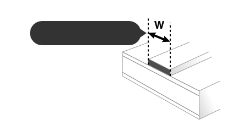# PCB Trace Width Calculator

This tool uses formulas from IPC-2221 to calculate the width of a copper printed circuit board conductor or "trace" required to carry a given current while keeping the resulting increase in trace temperature below a specified limit. If the length of the trace is also provided, the total resistance, voltage drop, and power loss due to trace resistance are also calculated. The results are estimates; actual results may vary depending on application conditions. Note also that required widths are significantly higher for traces on internal layers of a circuit board compared to those on a board’s outer surface; use whichever result is appropriate for your situation.

+More

Current
A
Thickness
Temperature Rise
Ambient Temperature
Trace Length

## Formula

This PCB Trace Width calculator uses formulas from IPC-2221.

Minimum Trace Width
mil### Internal Layers

Required Trace Width
=
Resistance
Ω =
Voltage Drop
V =
Power Loss
W =
Minimum Trace Width
mil### External Layers in Air

Required Trace Width
=
Resistance
Ω =
Voltage Drop
V =
Power Loss
W =